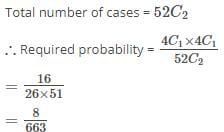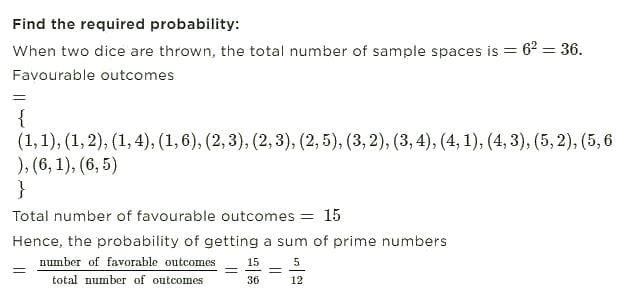UPSC  >  Test: Probability- 3

# Test: Probability- 3 - UPSC

Test Description

## 15 Questions MCQ Test CSAT Preparation - Test: Probability- 3

Test: Probability- 3 for UPSC 2023 is part of CSAT Preparation preparation. The Test: Probability- 3 questions and answers have been prepared according to the UPSC exam syllabus.The Test: Probability- 3 MCQs are made for UPSC 2023 Exam. Find important definitions, questions, notes, meanings, examples, exercises, MCQs and online tests for Test: Probability- 3 below.
Solutions of Test: Probability- 3 questions in English are available as part of our CSAT Preparation for UPSC & Test: Probability- 3 solutions in Hindi for CSAT Preparation course. Download more important topics, notes, lectures and mock test series for UPSC Exam by signing up for free. Attempt Test: Probability- 3 | 15 questions in 30 minutes | Mock test for UPSC preparation | Free important questions MCQ to study CSAT Preparation for UPSC Exam | Download free PDF with solutions
 1 Crore+ students have signed up on EduRev. Have you?
Test: Probability- 3 - Question 1

### A speaks the truth 3 out of 4 times, and B5 out of 6 times : what is the probability that they will contradict each other in stating the same fact?

Detailed Solution for Test: Probability- 3 - Question 1

Probability that A speak truth,P(A)=3/4

Probability that B speak truth,P(B)=5/6

Probability that A and B lie is
P(A′) lies=1/4​ P(B′) lies=1/6​
Required probability=P(A)×P(B′)+P(A′)×P(B)
=3/4​×1/6​+1/4​×5/6​
⇒8/24​⇒1/3

So, the correct option is B.

Test: Probability- 3 - Question 2

### Three groups of children contain respectively 3 girls & 1 boy; 2 girls & 2 boys; 1 girl & 3 boys. One child is selected at random from each group.The chance that the three selected children consists of one girl and 2 boys is

Detailed Solution for Test: Probability- 3 - Question 2

Let:
Group A: 3 girls, 1 boy
Group B: 2 girls, 2 boys
Group C: 1 girl, 3 boys
The combination of 1 girl and 2 boys can be selected in:
(i) A - girl, B - boy, C - boy: 3/4​×2/4​×3/4​=18/64

(ii) A - boy, B - girl, C - boy: 1/4*2/4*3/4 =6/64

(iii) A - boy, B - boy, C - girl: 1/4*2/4*1/4= 2/64.

Hence, required probability = 18/64+6/64+ 2/64 ​=26/64=13/32

So, the correct answer is c

Test: Probability- 3 - Question 3

### From a pack of 52 cards, two are drawn at random. Find the chance that one is a knave and the other a queen.

Detailed Solution for Test: Probability- 3 - Question 3Test: Probability- 3 - Question 4

Three coins are tossed. What is the probability of getting (i) neither 3 Heads nor 3 Tails?

Detailed Solution for Test: Probability- 3 - Question 4

(i) Probability of 3 heads = 1/8 Also, Probability of 3 tails =1/8.
Required probability = 1- (1/8 + 1/8) = 6/8 = 3/4.

Test: Probability- 3 - Question 5

Ram is visiting a friend. Ram knows that his friend has 2 children and 1 of them is a boy. Assuming that a child is equally likely to be a boy or a girl, then the probability that the other child is a girl, is

Detailed Solution for Test: Probability- 3 - Question 5

Total sample space ={B1​B2​,B1​G2​,G1​B2​}

Favourable ways ={B1​G2​,G1​B2​}

∴ Required probability =2/3.

So, the correct answer is C.

Test: Probability- 3 - Question 6

What is the probability of obtaining at least one tail when a coin is tossed five times?

Detailed Solution for Test: Probability- 3 - Question 6

‘At least one tail’ means that there can be one, two or three or four or five tails.
The only option that is not included is five heads.
The sum of all probabilities is always 1
P(at least one tail) = 1 - P(no tail)
P(no tail) = P(all heads) = P(H, H ,H, H ,H)
In any throw the probability of tail or head is ½
P(H, H ,H, H ,H) = 1/2 * 1/2 * 1/2 * 1/2 * 1/2       = (1/2)5
= 1/32
P( at least one tail ) : 1 - 1/32

Test: Probability- 3 - Question 7

Two fair dice are thrown. Find the probability of getting

(i) sum of numbers divisible by 2 or 4.

Detailed Solution for Test: Probability- 3 - Question 7

Positive outcomes are 2(1 way), 4(3 ways), 6(5 ways) 8(5 ways), 10 (3 ways), 12 (lway). Thus, 18/36= 1/2

Test: Probability- 3 - Question 8

Three cards numbered 2, 4 and 8 are put into a box. If a card is drawn at random, what is the probability that the card drawn is

(i) a prime number?

Detailed Solution for Test: Probability- 3 - Question 8

(i) Since 2 is the only prime number out of the three numbers, the answer would be 1/3

Test: Probability- 3 - Question 9

Two dice are thrown together. Then, the probability that the sum of numbers appearing on them is a prime number, is8.

Detailed Solution for Test: Probability- 3 - Question 9

The Correct Answer is D: 5/12Test: Probability- 3 - Question 10

If atleast one child in a family with 3 children is a boy then the probability that 2 of the children are boys, is

Detailed Solution for Test: Probability- 3 - Question 10

total no of ways are 8 but given that at least 1 boy is present so 1 case is excluded in which all three are girls
so total no ways in which at least one boy is present is 7
total no ways in which 1 girl and 2 boys are present are 3 ways
so the probability is 3/7

So, the correct option is A.

Test: Probability- 3 - Question 11

In rolling two dices, find the probability (i) there is at least one ‘6’

Detailed Solution for Test: Probability- 3 - Question 11

(i) With a six on the first dice, there are 6 possibilities of outcomes that can appear on the other dice (viz. 6 & 1, 6 & 2, 6 & 3, 6 & 4, 6 & 5 and 6 & 6). At the same time with 6 on the second dice there are 5 more possibilities for outcomes on the first dice: (1 & 6 , 2 & 6 , 3 & 6, 4 & 6, 5 & 6)
Also, the total outcomes are 36. Hence, the required probability is 11/36.

Test: Probability- 3 - Question 12

Amit throws three dice in a special game of Ludo. If it is known that he needs 15 or higher in this throw to win then find the chance of his winning the game.

Detailed Solution for Test: Probability- 3 - Question 12

Event definition is: 15 or 16 or 17 or 18. 15 can be got as: 5 and 5 and 5 (one way) Or 6 and 5 and 4 (Six ways) Or 6 and 6 and 3 (3 ways) Total 10 ways. 16 can be got as: 6 and 6 and 4 (3 ways) Or 6 and 5 and 5 (3 ways) Total 6 ways. 17 has 3 ways and 18 has 1 way of appearing. Thus, the required probability is:(10 + 6 + 3 + l)/216 = 20/216 = 5/54.

Test: Probability- 3 - Question 13

In a horse race there were 18 horses numbered 1-18. The probability that horse 1 would win is 1/6, that 2 would win is 1/10 and that 3 would win is 1/8.
Assuming that a tie is impossible, find the chance that one of the three will win.

Detailed Solution for Test: Probability- 3 - Question 13

1/6 + 1/10 + 1/8 = 47/120

Test: Probability- 3 - Question 14

Two fair dice are thrown. What is the probability of (i) throwing a double?

Detailed Solution for Test: Probability- 3 - Question 14

(i) There are six doubles (1, 1; 2 & 2; 3 & 3; 4 & 4; 5 & 5; 6 & 6) out of a total of 36 outcomes

6/36 = 1/6

Test: Probability- 3 - Question 15

How many words, no matter if they are meaningless, can be formed by the letters of the word 'DIARY'?

Detailed Solution for Test: Probability- 3 - Question 15

The correct answer is None of the above.
Key Points

Total number of letters = 5

Number of repeating letters = 0

The total number of words that can be formed are = 5P5

= 5!

= 120

∴The total number of words that can be formed is 120.
Alternate Method

• There are 5 different letters in the word DIARY.
• The first place can be filled in 5 ways.
• The second place can be filled by any one of the remaining 4 letters. So, second place can be filled in 4 ways.
• So, on continuing, the number of ways of filling third place in 3 ways, fourth place in 2 ways, fifth place in 1 way.
• Therefore, the number of words that can be formed using all the letters of the word DIARY, using each letter exactly once is 5×4×3×2×1 = 5! = 120

## CSAT Preparation

197 videos|151 docs|200 tests
Information about Test: Probability- 3 Page
In this test you can find the Exam questions for Test: Probability- 3 solved & explained in the simplest way possible. Besides giving Questions and answers for Test: Probability- 3, EduRev gives you an ample number of Online tests for practice

## CSAT Preparation

197 videos|151 docs|200 tests

### How to Prepare for UPSC

Read our guide to prepare for UPSC which is created by Toppers & the best Teachers